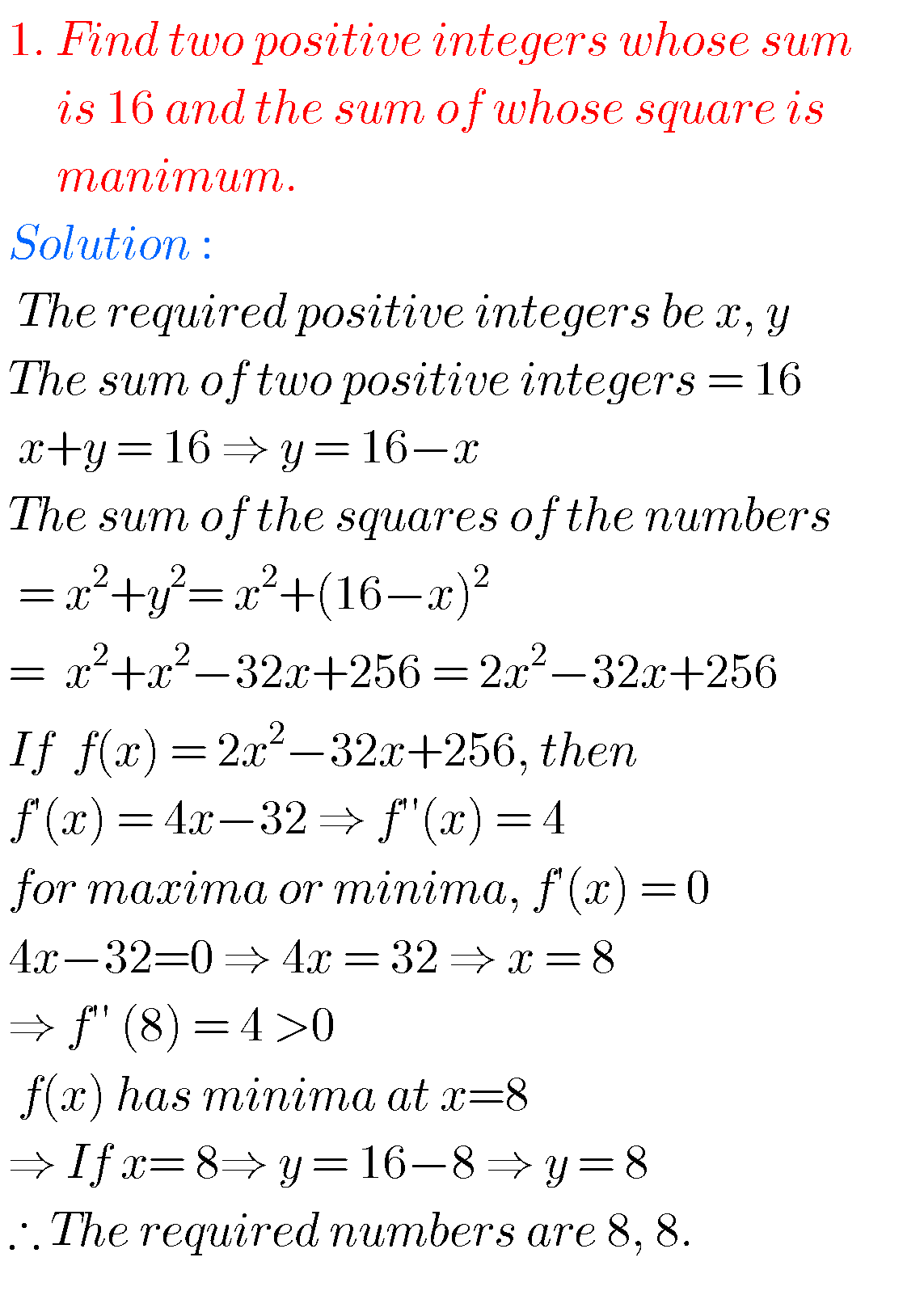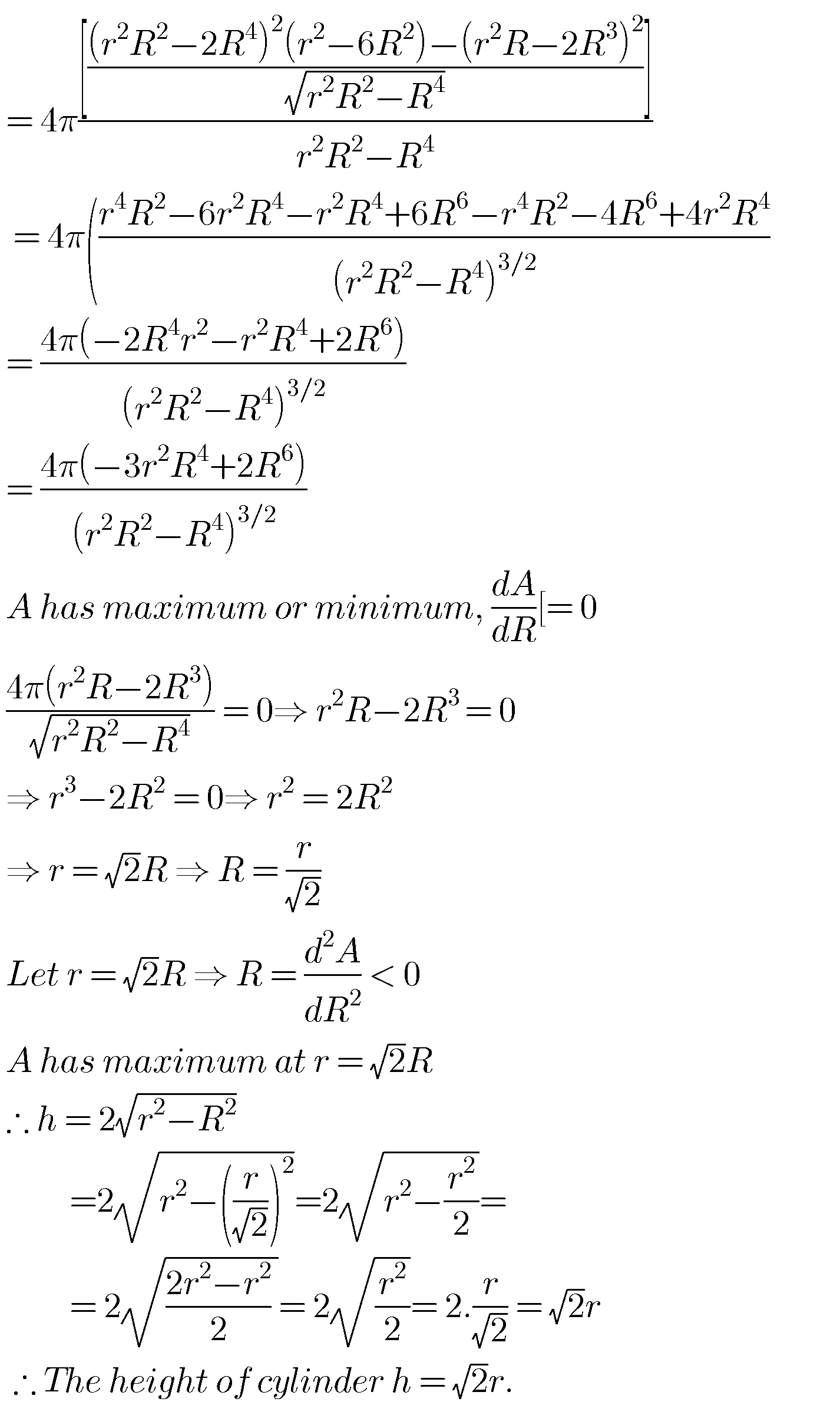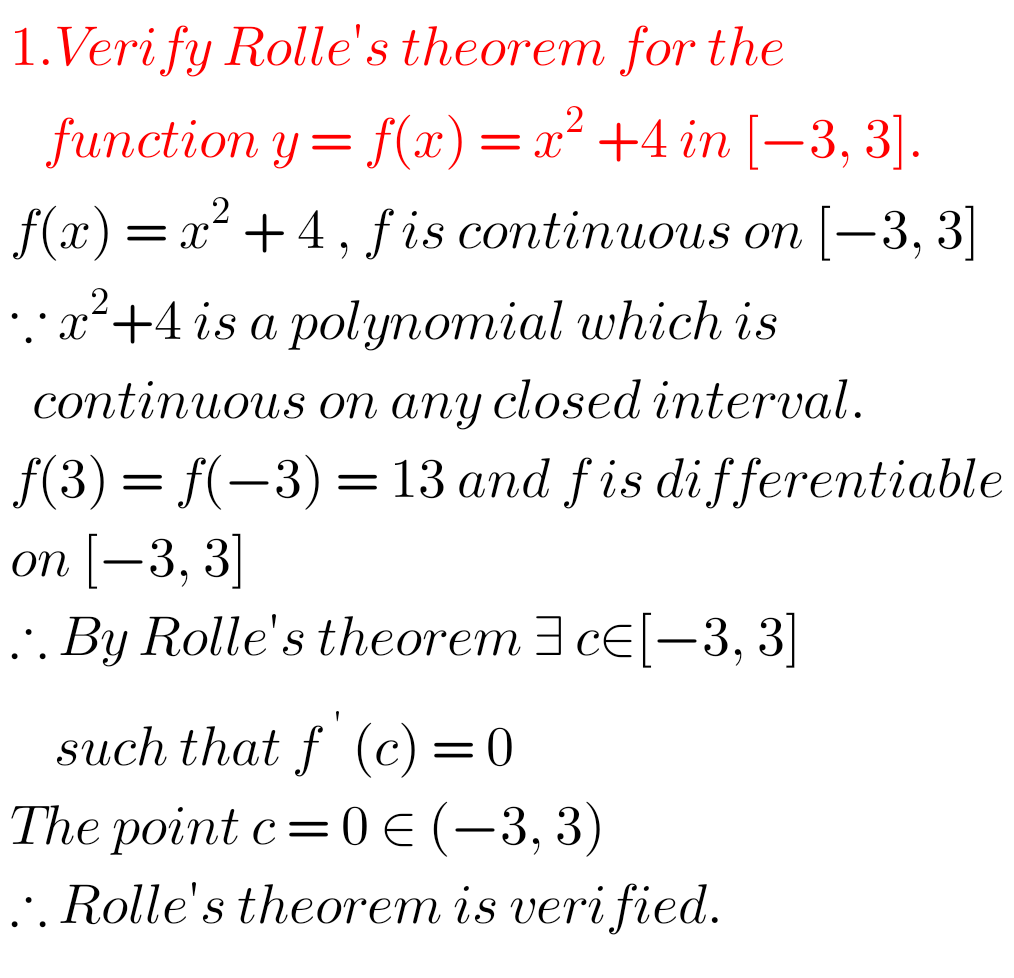# Maxima and minima, Intermediate maths 1b solutions

Inter maths 1b solutions for some problem for Maxima and minima (10.5) are given.

These are very easy to understand. First you study the text book very well.

Observe the solutions and try them in your own method.

Text book exercise solutions links are also given.

Applications of Derivatives

Exercise 10(a)

Exercise 10(b)

Exercise 10(c)

Exercise 10(d)

Exercise 10(e)

Exercise 10(f)

Exercise 10(g)

Exercise 10(h)

You can also see

Inter Maths 1A textbook solutions

Inter Maths 1B textbook solutions

Inter Maths IIA textbook solutions

Inter Maths IIB textbook solutions

M

## Intermediate mathematics 1b chapter 10.5 solutions

Maxima and MinimaNote – Observe the solutions and try them in your own methods.You can see the solutions for junior inter maths 1b

1. Locus

Straight lines sa

11. Rate measure

Inter maths trigonometry solutions

Maths real numbers solutions class 10

### 1 thought on “Maxima and minima,Inter maths 1b solutions”

1.If x,y,z are length of the perpandicular dropped from a point inside the triangle of given area A,on the three sides of the triangle then the minimum value of x^2+y^2+Z^2 is 4A^2/a^2+b^2+c^2